2

# Calculating Moving Averages in Pyramid IMDB

## Introduction

When looking at trends over time, there is often the need to smooth out the individual values by using a Moving Average (MAv). The MAv smooths out the peaks and troughs, based on averaging a defined number of past time periods.

## Approach

It would be great to be able to take the current time period, then select a number of periods back to specified point, then use those two points to define a Range. However, currently in Pyramid, when using the In Memory DataBase or other SQL based databases, the CurrentMember() function does not actually return the member name and so cannot be used as an endpoint in a Range() function. We hope to add this functionality in a future release.

However, you can achieve MVa calculations by using multiple instances of the Lag() function in your calculation. Here's an example of a 3 month rolling average. The first Data Point defines the measure, sales, and the Current Member of the time period concerned, in this case months. Subsequent DataPoints use the Lag() function on the months, incrementing the lag by one each time, so that the last DataPoint is, in this instance Lag(2). We add these together, then divide by 3: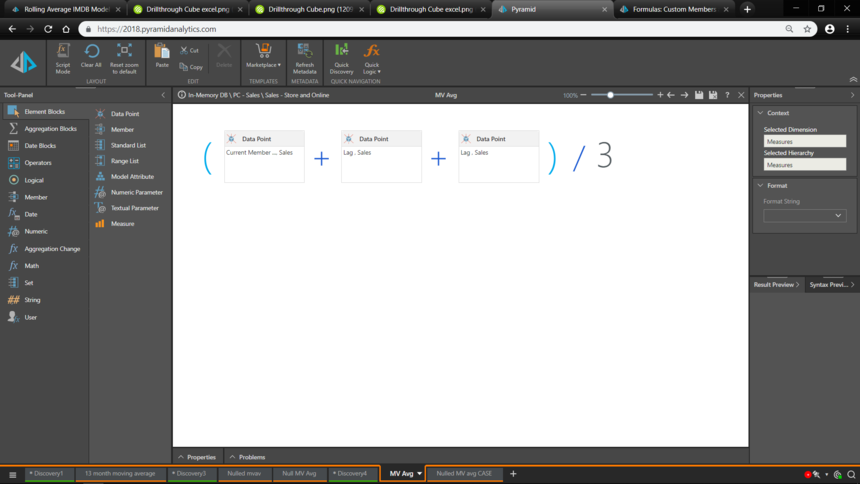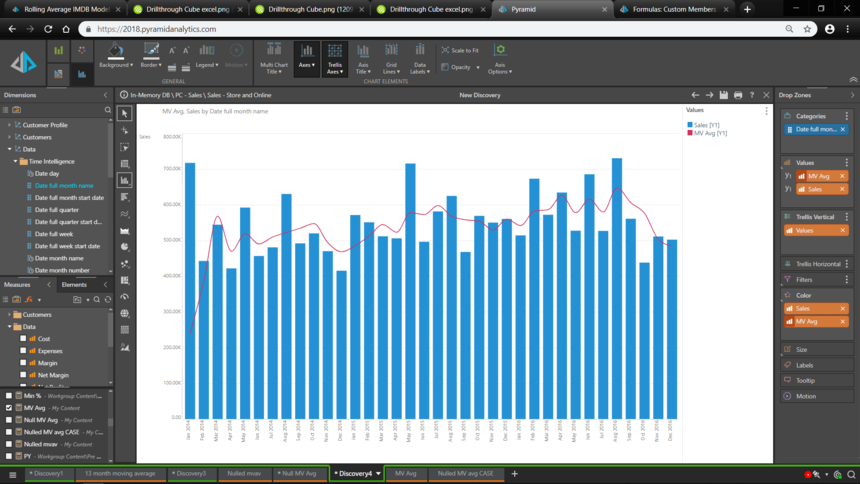However, the first and second values for my 3 month MVa are low because there are only 1 and 2 time periods available respectively and my average is always dividing by 3. This may be what you want, but usually in MVa calculations, if there are insufficient elements available in the SUM for the  denominator, the average is zeroed or nulled.

We can achieve this by adding an IF statement, testing for nulls for Sales in the LAG(2) time periods. If there is no time period at LAG(2), then it follows that there will not be 3 time periods available, so we do not need to test further.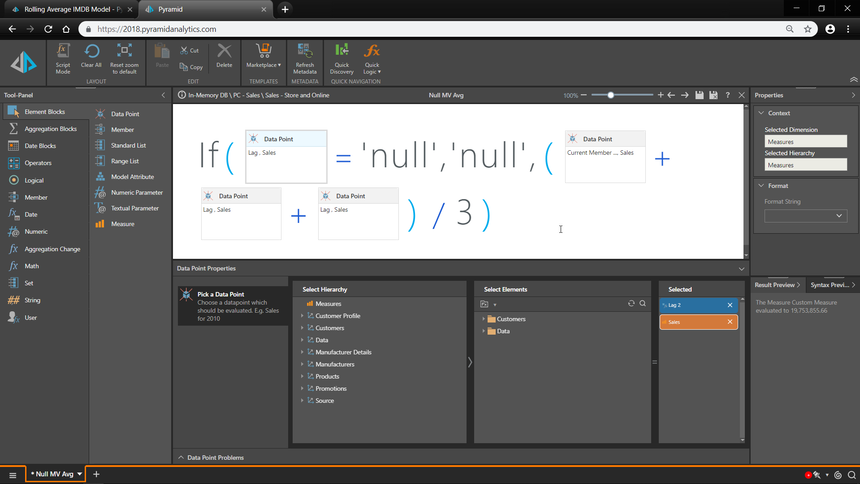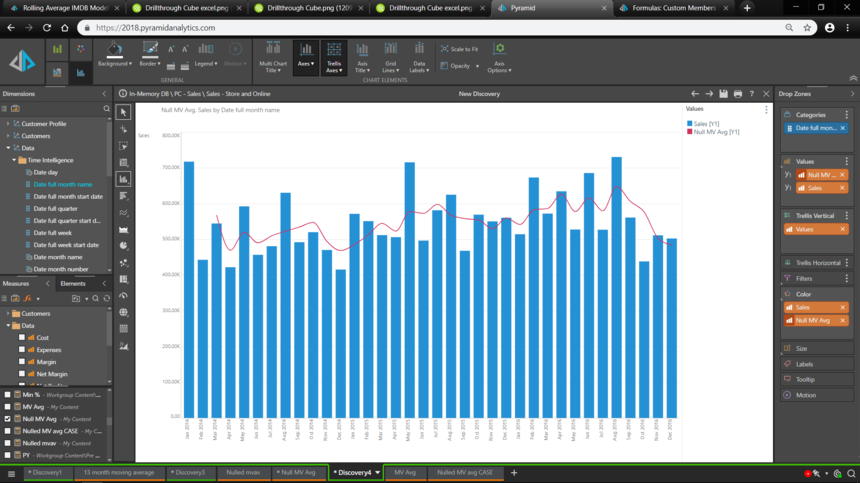Here's a 13 month moving average using the same technique: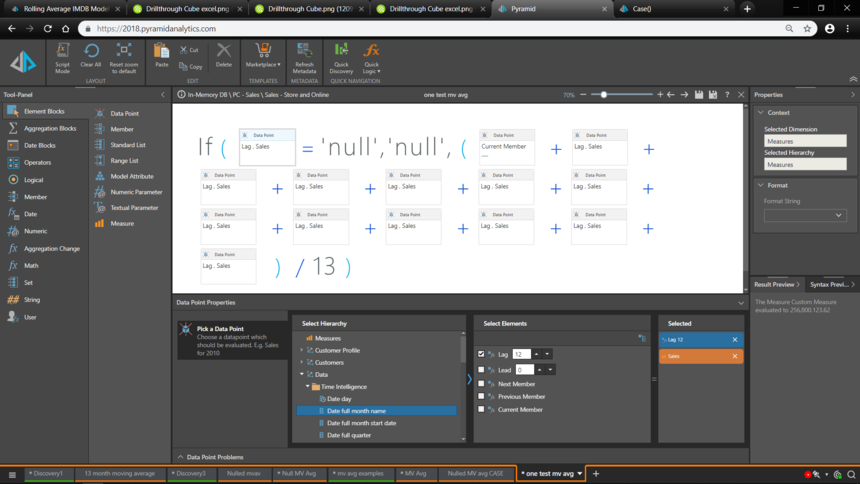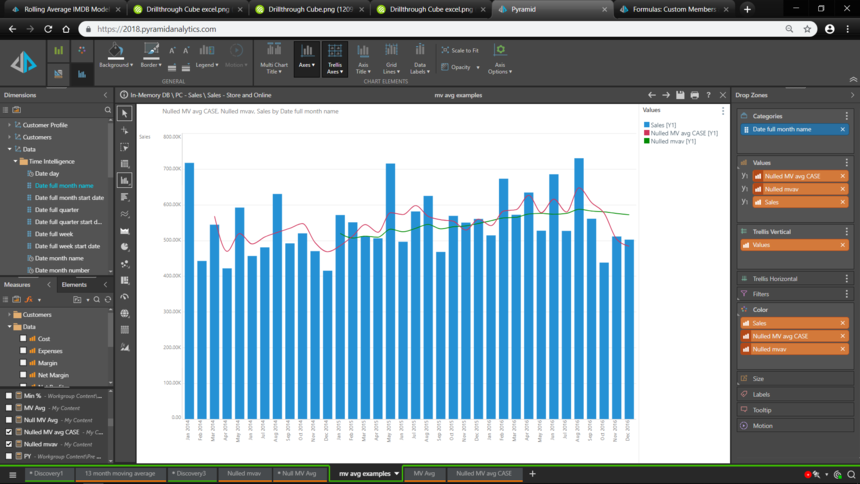## Conclusions

Moving Averages are a very common analytic technique. By using the LAG() function and some testing on nulls, they can be achieved easily and simply against model data stored in the Pyramid IMDB or any other database.

Ian

## Note

If you are using SQLServer Analysis Services as the underlying data source, then the RANGE() function can be used, since SSAS MDX CurrentMember() function DOES return the member name and hence the RANGE() function will return the set of time periods from the current member back to whatever LAG() value you specify as the starting point.